Site Overlay

# 电路基础笔记：第一章 电路模型和电路定律

【例子】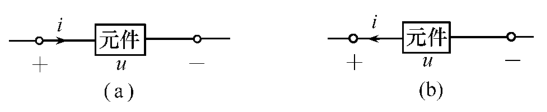(1) u, i 的参考方向是否关联?

(2) u, i 的乘积表示什么功率?

(3) 若(a)图 $u>0, i<0$, (b) 图 $u>0, i>0$, 元件是发出还是吸收功率?

## 关联参考方向

$+-$表示电势, 箭头表示电流方向:

$+\to-$ 或者 $-\leftarrow +$ 就是关联参考方向.

$-\to+$ 或者 $+\leftarrow-$就是非参考关联方向.

## 吸收功率和发出功率，功率平衡

$P = k\cdot UI$ 其中 $k$ 表示是否关联, 是取 $1$, 不是取 $-1$. 如果 $P>0$ 就是在消耗能量, 吸收功率. 反之则是提供能量, 发出功率. 当一个完整电路的所有元件，吸收功率等于发出功率时，称其功率平衡

(3) (a) 中 $P = k\cdot UI = 1\times ui < 0$ 发出功率.(b) 中 $-1ui<0$ 发出功率.

【例子】写出下面各个元件的$u,i$约束方程：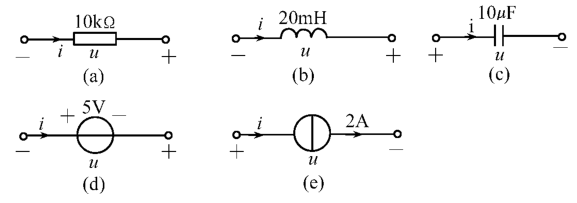## 约束方程

$u,i$ 约束方程其实就是 $u, i$ 之间的关系式(注意参考方向!!影响正负). 当然根据原件不同会牵扯进其它物理量, 比如时间 $t$.

## 常见元件

### 电阻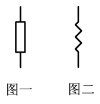### 电感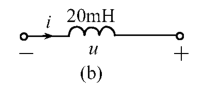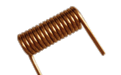$$\Psi = N \phi$$

N 是匝数也就是有几个圈圈.

$E_L = k \frac{dI}{dt}$ 这个比例系数和线圈本身相关, 所以就拿来定义了物理量自感 $L$:

$$E_L = -L\frac{dI}{dt}$$### 电容

$$C = \frac{Q}{U}$$

$$i = C \frac{du}{dt}$$

(下面均指理想恒流源恒压源)

### 电流源

• 电流源内阻无穷大
• 电流源提供电流
• 内阻无穷大的电路电流为 0## 功率平衡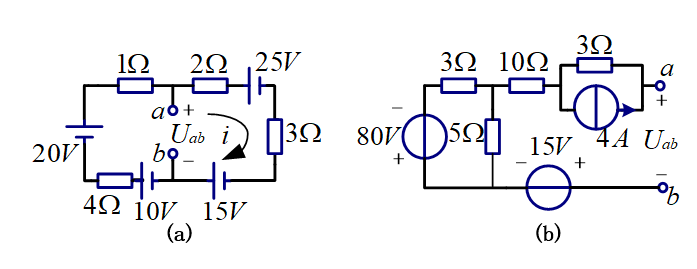## 复杂电路求电势方法, 基尔霍夫定律

(a) 我们要求 ab 之间的电压, 就要知道 a 点电势和 b 点电势; 要求电势, 就要知道电流; 要求电流, 就要知道总电压; 如何求总电压?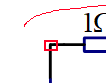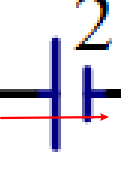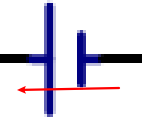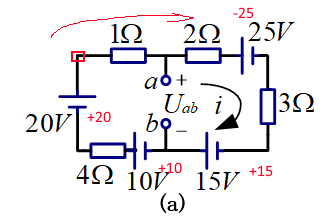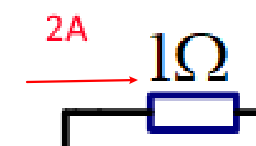2A 的电流流过这个 $1\Omega$的电阻, 电压降低了 $u_1 = 2A \cdot 1 \Omega = 2V$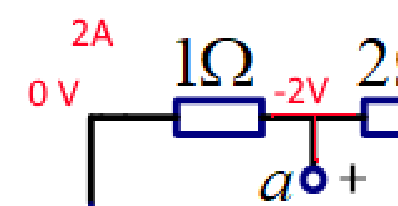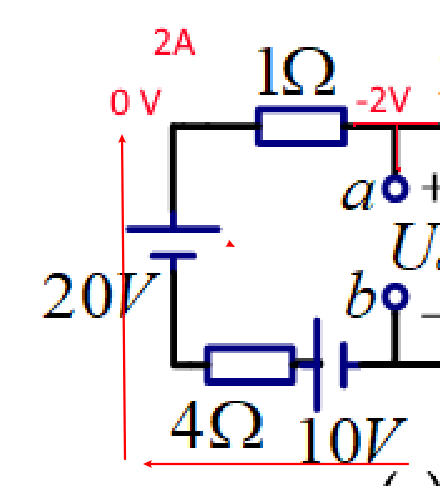$u_{ab} = u_a - 0 = u_a = 0 + 10V - 8V + 20V - 2V = 20V$ ,结果是一样的: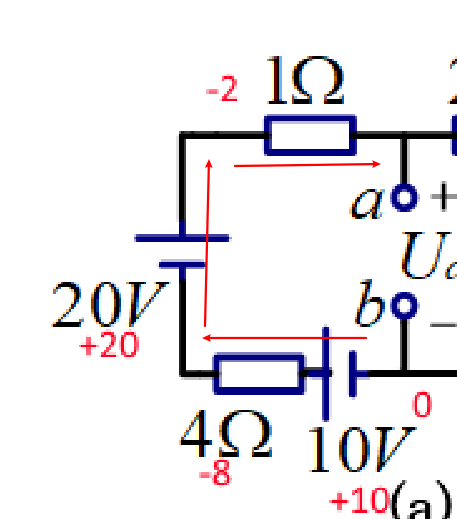## 电流源和电压源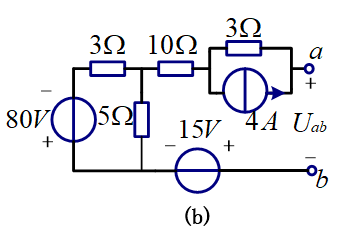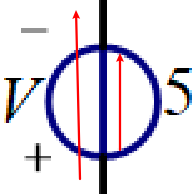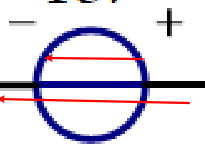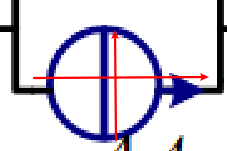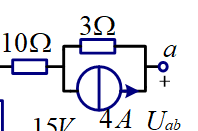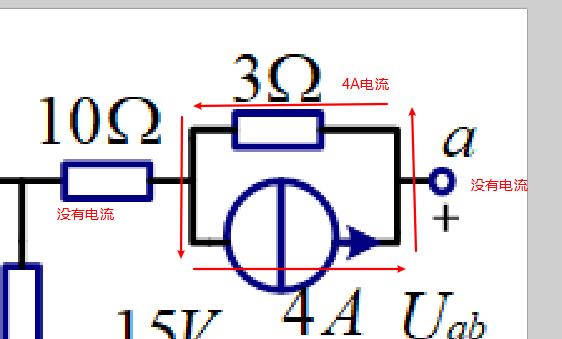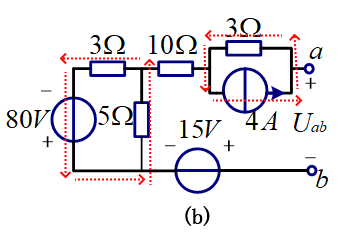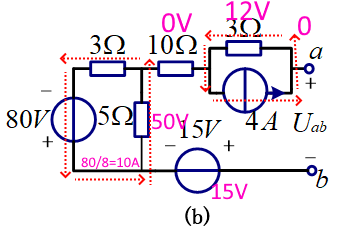$0 - 15V - 50V +0V + 12V = u_a = -53V$